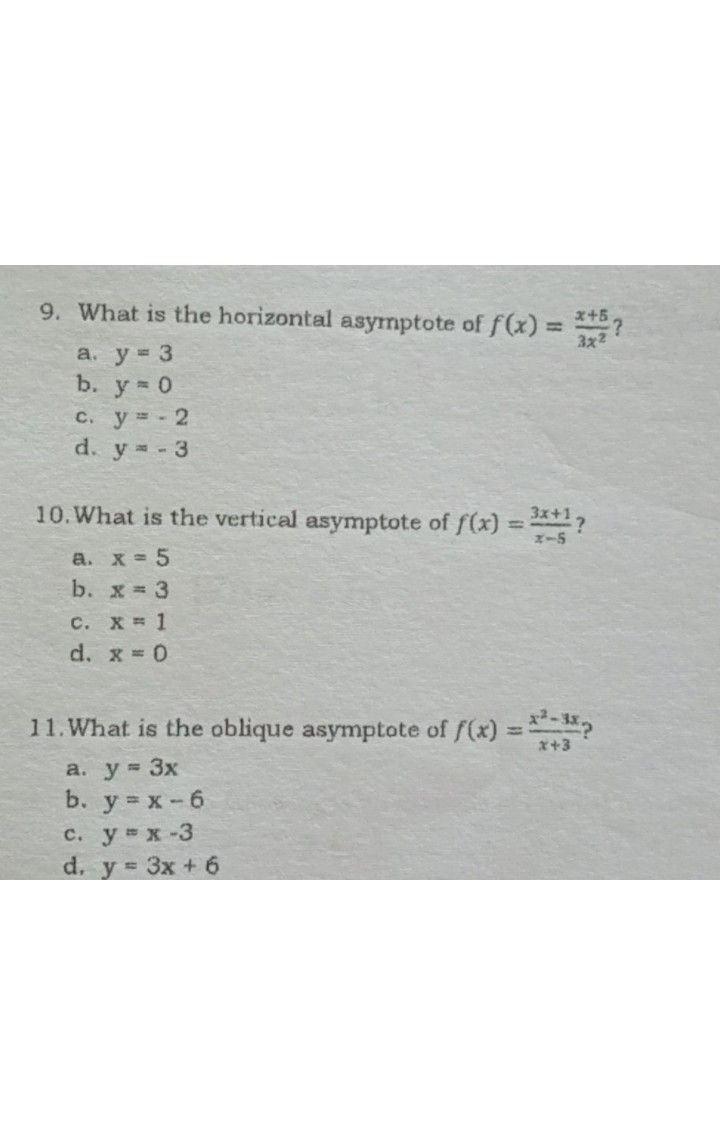Symbol
Problem8. If the degree of the leading coefficient of the numerator is equal to the degree of the leading coefficient of the denominator ofa rational function, which of the following statements has to be true? a. The graph has no asymptote b. The graph of the function has slant asymptote c. The graph of the function has a horizontal asymptote d. None of the above 9. What is the horizontal asyrnptote of $f\left(x\right)=$ $=\dfrac {x2} {7x^{x}}7$ a. $y=3$ b. $y=0$ C. y - 2 d. $y=-3$ 10.What is the vertical asymptote of $f\left(x\right)=$ ? a. $x=5$ b. $x=3$ C. X 1 $x=0$ d. 11.What is the oblique asymptote of $f\left(x\right)=\dfrac {x^{2}.1x} {x+3}2$ a. $y=3x$ b. $y=x-6$ c. $y=x-3$ d. $y=3x+6$ 12. Oblique asymptote occurs when there is no horizontal asymptote, the statement is a. Always true b. Sometimes true c. Never true d. Cannot be determined 13. How will you describe the horizontal asymptote $off\left(x\right)=\dfrac {3} {3+x}2$ a. does not exist b. approaching at $x=3$ c. approaching at $y=-3$ d. approaching $aty=0$
10th-13th grade
Probability and Statistics
Search count: 107
SolutionQanda teacher - chandan
can you ask individual questionsStudentcan you help me?Qanda teacher - chandan
yeah i'm trying to solve itStudent
yes pls help me I have you 5 star
I gave*Qanda teacher - chandan
yeah ok i'm not getting accurate answe